# High School Chemistry : Phases of Matter

## Example Questions

### Example Question #5 : Using Boyle's Law

A sample of oxygen gas has a volume of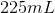when its pressure is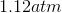. What will the volume of the gas be at a pressure of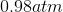if the temperature remains constant?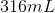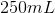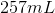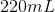Explanation:

To solve this question we will need to use Boyle's law: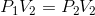We are given the initial pressure and volume, along with the final pressure. Using these values, we can calculate the final volume.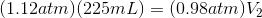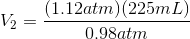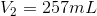### Example Question #6 : Using Boyle's Law

A gas is initially in a 5L piston with a pressure of 1atm.

If pressure changes to 3.5atm by moving the piston down, what is new volume?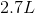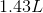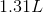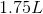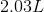Explanation:

Use Boyle's Law: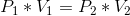Plug in known values and solve for final volume.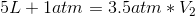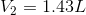### Example Question #1 : Using Boyle's Law

A balloon of volume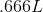at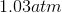is placed in a pressure chamber where the pressure becomes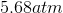, determine the new volume.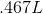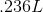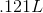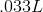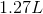Explanation:

Use Boyle's law and plug in appropriate parameters: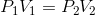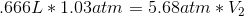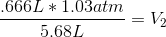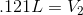### Example Question #1 : Using Boyle's Law

A gas in a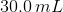container is at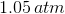is compressed to a volume of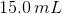. What is the new pressure of the container?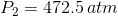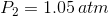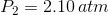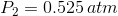Explanation:

Boyle's Law is:The initial volume (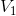) and pressure (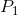) of the gas is given. The volume changes to a new volume (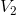). Our goal is to find the new pressure (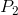). Solving for the new pressure gives: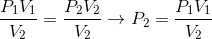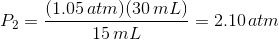Notice the answer has 3 significant figures.

### Example Question #1 : Using Gay Lussac's Law

What happens to the pressure of a closed gaseous system when the temperature increases two-fold?

The pressure is quartered

The pressure stays the name

The pressure is halved

The pressure is doubled

The pressure is doubled

Explanation:

To solve this question, we will need to use Gay-Lussac's Law: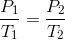This law shows a direct relationship between temperature and pressure: as temperature increases, pressure will increase proportionally. Mathematically, we can solve for the change in pressure by inputting the change in temperature. The second temperature is equal to twice the first temperature. For the fractions to remain equal, the second pressure must also be twice the first pressure.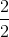### Example Question #1 : Using Gay Lussac's Law

An ideal gas is contained in a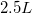container at a pressure of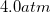. The container is at a temperature of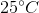.

What will be the final pressure if the temperature is increased to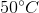?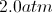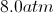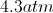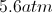Explanation:

Since the only variable that has changed is temperature, we can use Gay-Lussac's law in order to compare pressure to temperature. Because temperature and pressure are on opposite sides of the ideal gas law, they are directly proportional to one another. In other words, as one increases, the other increases as well.

Gay-Lussac's law is written as follows:To use this equation, we must convert the temperature to Kelvin.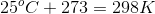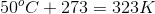Now, use these temperatures and the initial pressure to solve for the final pressure.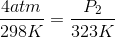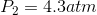### Example Question #3 : Using Gay Lussac's Law

What law is represented by the following formula?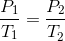Combined gas law

Boyle's law

Ideal gas law

Gay-Lussac's law

Charles's law

Gay-Lussac's law

Explanation:

Gay-Lussac's law defines the direct relationship between temperature and pressure. When the parameters of a system change, Gay-Lussac's law helps us anticipate the effect the changes have on pressure and temperature.Boyle's law relates pressure and volume:Charles's law relates temperature and volume: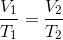The combined gas law takes Boyle's, Charles's, and Gay-Lussac's law and combines it into one law: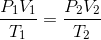The ideal gas law relates temperature, pressure, volume, and moles in coordination with the ideal gas constant: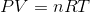### Example Question #1 : Using Gay Lussac's Law

The graph depicted here represents which of the gas laws?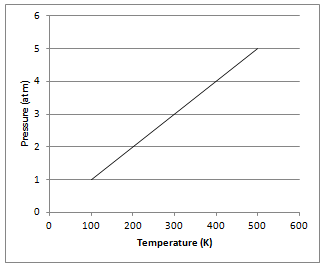Boyle's law

Newton's second law

Charles's law

Gay-Lussac's law

Gay-Lussac's law

Explanation:

The graph shows that gas pressure varies directly with Kelvin temperature at a constant volume, as determined by Gay-Lussac. Gay-Lussac's law can be represented mathematically for a given mass of gas at a constant volume as follows:Boyle's law shows the relationship between pressure and volume. Charles's law shows the relationship between volume and temperature. Newton's second law is not related to gases and shows the relationship between force, mass, and acceleration.

### Example Question #1 : Using Gay Lussac's Law

A family leaves for summer vacation by driving on the highway. The car’s tires start the trip with a pressure of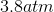at a temperature of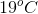and a volume of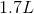. What is the pressure of the tires after driving, when the temperature within the tire increases to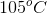?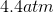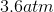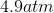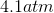Explanation:

We can assume that the volume of the tires remains constant. This allows us to apply Gay-Lussac's law, which related pressure and temperature:We know both the initial and final temperatures, but we must convert to Kelvin in order to use SI units.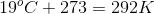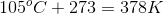Using these temperatures and the initial pressure, we can solve for the final pressure.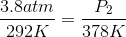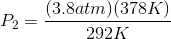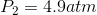### Example Question #6 : Using Gay Lussac's Law

If the temperature of a gas increases, what happens to the pressure?

Pressure will increase

Pressure will decrease

We must know the volume of the gas to determine the relationship

We must know the molecular formula of the gas to determine the relationship

Pressure will remain unchanged